Select Page

# CBSE MCQ in English Solutions for Maths 12 Science Continuity and Differentiability

CBSE MCQ in English Solutions for Maths 12 Science Continuity and Differentiability to enable students to get Solutions in a narrative video format for the specific question.

Expert Teacher provides CBSE MCQ Solutions for Maths 12 Science Continuity and Differentiability through Video Solutions in English language. This video solution will be useful for students to understand how to write an answer in exam in order to score more marks. This teacher uses a narrative style for a question from Continuity and Differentiability not only to explain the proper method of answering question, but deriving right answer too.

Please find the question below and view the Solution in a narrative video format.

Question:

Solution Video in English:

You can select video Solutions from other languages also. Please check Solutions in ( Hindi )

## Similar Questions from CBSE, 12th Science, Maths, Continuity and Differentiability

Question 1 : Findfor the function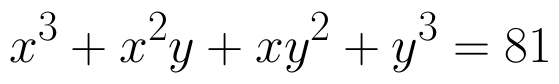. (View Answer Video)

Question 2 : Differentiate the function w.r.t.x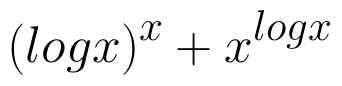. (View Answer Video)

Question 3 : If x and y are connected parametrically by the equation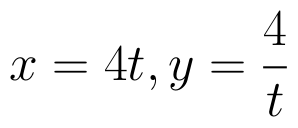, without eliminating the parameter, find. (View Answer Video)

Question 4 :  Find the second order derivative of the function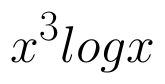. (View Answer Video)

Question 5 : Differentiate the function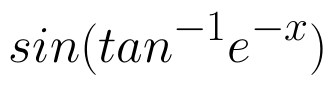with respect to x. (View Answer Video)

### Linear Programming

Question 1 : The objective function is maximum or minimum, which lies on the boundary of the feasible region. (View Answer Video)

### Inverse Trigonometric Functions

Question 1 : If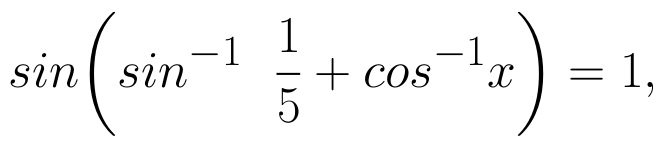then find the value of x. (View Answer Video)

Question 2 : Write the principal value of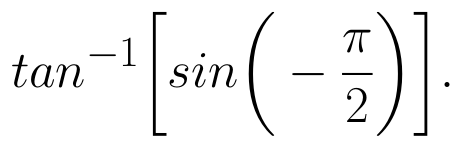(View Answer Video)

Question 3 : Write the value of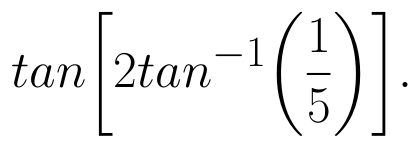(View Answer Video)

Question 4 :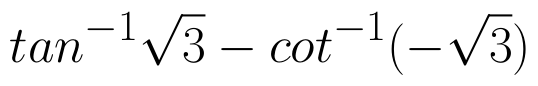is equal to : (View Answer Video)

Question 5 : Write the value of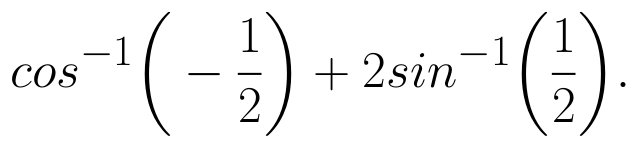(View Answer Video)

### Application of Integrals

Question 1 : Using the method of integration, find the area of the region bounded by the lines: 5x - 2y -10 = 0, x + y - 9 = 0 and 2x - 5y - 4 = 0. (View Answer Video)

Question 2 : Using integration, find the area of the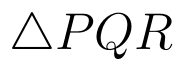co-ordinates whose vertices are P(2,0), Q(4, 5) and R(6,3). (View Answer Video)

Question 3 : Area lying in the first quadrant and bounded by the circle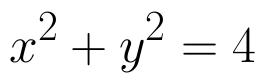and the lines x =0 and x = 2 is (View Answer Video)

Question 4 : Find the area of the region bounded by the curve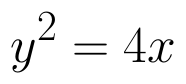and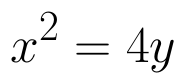. (View Answer Video)

Question 5 : Using the method of integration find the area of the triangle ABC, coordinates of whose vertices are A(2, 0), B(4, 5) and C(6, 3). (View Answer Video)